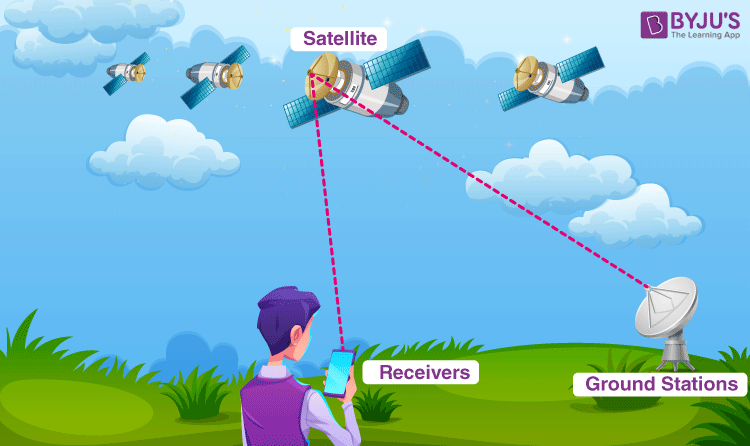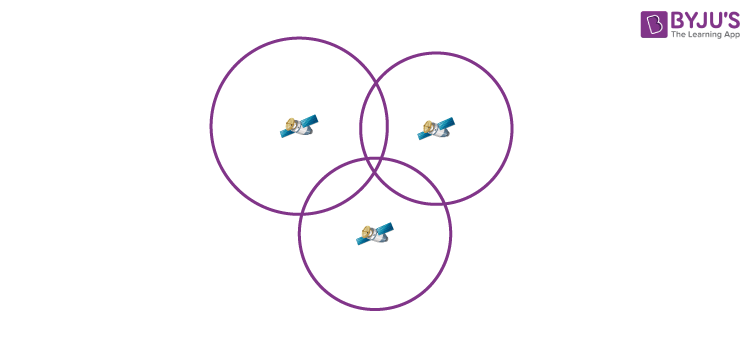Checkout JEE MAINS 2022 Question Paper Analysis : Checkout JEE MAINS 2022 Question Paper Analysis :

# What is GPS (Global Positioning System)

Ancient sailors used the constellations in the night sky to reckon where they were and where they were going. Likewise, humans have relied on the skies to find their way since ancient times. Now, all we need is a simple hand-held GPS (short for Global Positioning System) receiver to comprehend precisely where we are anywhere in the world. But we still require objects high in the sky to figure out where we are and how to get to other places.

## What is GPS?

GPS stands for Global Positioning System. It is a radio navigation system used in land, sea, and air to determine the exact location, time and velocity irrespective of weather conditions. The US military first used it in the year 1960.

### Components of a GPS system

GPS is a system and it is made up of three parts: satellites, ground stations, and receivers.Following are the functionalities of each of these parts:

• Satellites act like the stars in constellations, and we know where they are because they invariably send out signals.
• The ground stations make use of the radar to make sure the satellites are actually where we think they are.
• A receiver is a device that you might find in your phone or in your car and it constantly seeks for the signals from the satellites. The receiver figures out how far away they are from some of them. Once the receiver calculates its distance from four or more satellites, it knows exactly where you are.

## How GPS Works?

There are at least 4 GPS satellites in the line of sight of a receiver on the earth. The transmitter GPS sends information about the position and time to the receiver GPS at fixed intervals. The signals that are sent to the receiver devices are radio waves. By finding the difference in time between the signal sent from the GPS satellite to the time the GPS receives, the distance between the GPS receiver and the satellite can be calculated. Using the trilateration process, the receiver locates its position as the signals are obtained from at least three satellites.

For a GPS to calculate a 2-D position, which includes the latitude and longitude, a minimum of 3 satellites are required. For a 3-D position that provides latitude, longitude, and altitude, a minimum of 4 satellites are needed.

### Quick Summary

 How Does GPS work? GPS is a system of 30+ navigation satellites orbiting the Earth. We know their location precisely because they invariably send out signals. The GPS receiver in your phone receives these signals. Once the receiver calculates its distance from four or more GPS satellites, it can figure out exactly where you are.

## What Is Trilateration?Trilateration is defined as the process of determining the location based on the intersections of the spheres. The distance between the satellite and the receiver is calculated by considering a 3-D sphere such that the satellite is located at the centre of the sphere. Using the same method, the distance for all the 3 GPS satellites from the receiver is calculated.

Following are the parameters that are calculated after trilateration:

• Time of sunrise and the sunset
• Speed
• Distance between the GPS receiver to the destination

GPS systems are remarkably versatile and can be found in almost any industry sector. They can be used to map forests, help farmers harvest their fields and navigate aeroplanes on the ground or in the air.

## Frequently Asked Questions – FAQs

### What is GPS?

GPS or Global Positioning System is a radio navigation system used in land, sea, and air to determine the exact location, time and velocity irrespective of weather conditions.

### What are the three components of GPS?

GPS is made of three components namely, satellites, ground stations, and receivers.

### How does GPS work?

By finding the difference in time between the signal sent from the GPS satellite to the time the GPS receives, the distance between the GPS receiver and the satellite can be calculated. Using the trilateration process, the receiver locates its position as the signals are obtained from at least three satellites.

### What is Trilateration?

Trilateration is defined as the process of determining the location based on the intersections of the spheres.

### Name the parameters that are calculated after trilateration.

The following parameters are calculated after the trilateration: a) Time of sunrise and the sunset b) Speed c) Distance between the GPS receiver to the destination.